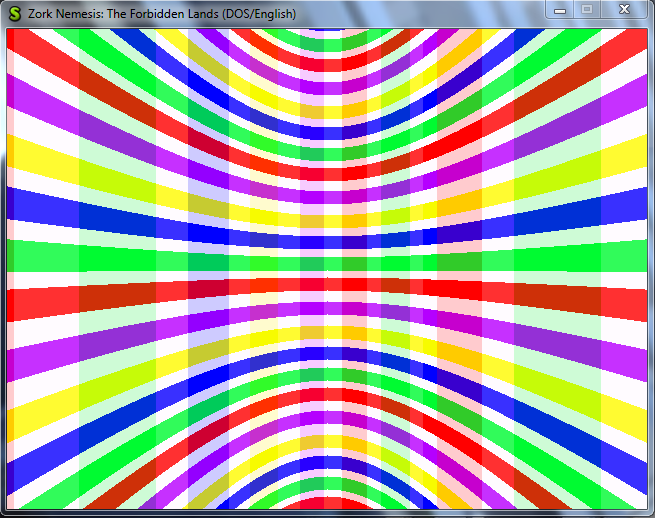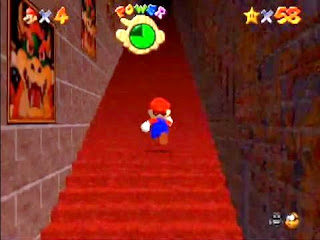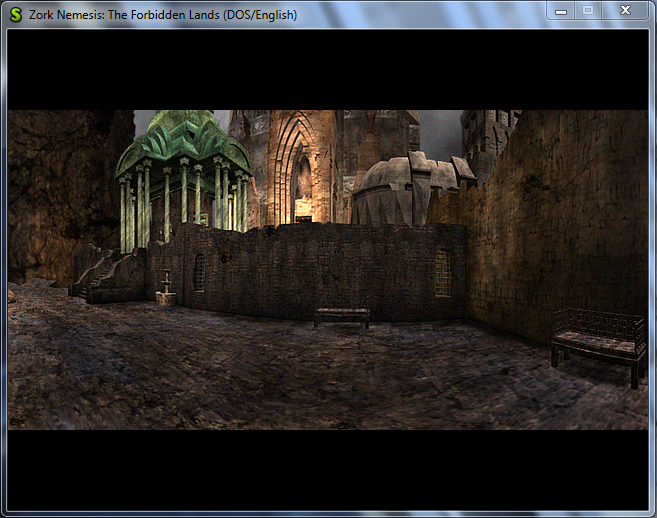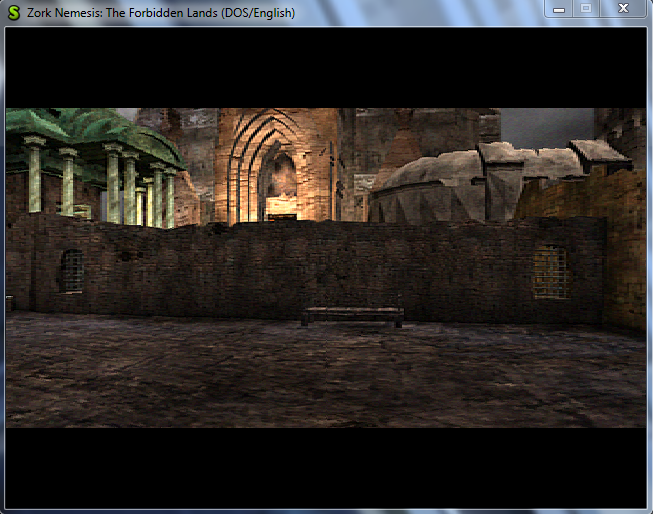## Saturday, August 3, 2013

### The making of psychedelic pictures (AKA, the panorama system)In the game, the backgrounds are very long 'circular' images. By circular, I mean that if you were to put two copies of the same image end-to-end, they would be continuous. So, when the user moves around in the game, we just scroll the image accordingly. However, being that the images are flat, this movement isn't very realistic; it would seem like you are continually moving sideways through an endless room. (Endless staircase memories anyone?)To counter this, the makers of ZEngine created 'ZVision': they used trigonometry to warp the images on the screen so, to the user, it looked like you were truly spinning 360 degrees. So let's dive into how exactly they did that.

The basic premise is mapping an image onto a cylinder and then mapping it back onto a flat plane. The math is all done once and stored into an offset lookup table. Then the table is referenced to warp the images.Without warpingWith warping

You'll notice that the images are pre-processed as though they were captured with a panorama camera.

Video example:

Here is the function for creating the panorama lookup table:
void RenderTable::generatePanoramaLookupTable() {
memset(_internalBuffer, 0, _numRows * _numColumns * sizeof(uint16));

float halfWidth = (float)_numColumns / 2.0f;
float halfHeight = (float)_numRows / 2.0f;

float fovRadians = (_panoramaOptions.fieldOfView * M_PI / 180.0f);
float halfHeightOverTan = halfHeight / tan(fovRadians);
float tanOverHalfHeight = tan(fovRadians) / halfHeight;

for (uint x = 0; x < _numColumns; x++) {
// Add an offset of 0.01 to overcome zero tan/atan issue (vertical line on half of screen)
float temp = atan(tanOverHalfHeight * ((float)x - halfWidth + 0.01f));

int32 newX = int32(floor((halfHeightOverTan * _panoramaOptions.linearScale * temp) + halfWidth));
float cosX = cos(temp);

for (uint y = 0; y < _numRows; y++) {
int32 newY = int32(floor(halfHeight + ((float)y - halfHeight) * cosX));

uint32 index = y * _numColumns + x;

// Only store the x,y offsets instead of the absolute positions
_internalBuffer[index].x = newX - x;
_internalBuffer[index].y = newY - y;
}
}
}


I don't quite understand all the math here, so at the moment it is just a cleaned-up version of what Marisa Chan had. If any of you would like to help me understand/clean up some of the math here I would be extremely grateful!

Putting aside the math for the time being, the function creates an (dx, dy) offset at each (x,y) coordinate. Or in other words, if we want the pixel located at (x,y), we should instead look at pixel (x + dx, y + dy). So to blit an image to the screen, we do this:
1. Iterate though each pixel
2. Use the (x,y) coordinates to look up a (dx, dy) offset in the lookup table
3. Look up that pixel color in the source image at (x + dx, y + dy)
4. Set that pixel in the destination image at (x,y)
5. Blit the destination image to the screen using OSystem::copyRectToScreen()

Steps 1 - 4 are done in mutateImage()
void RenderTable::mutateImage(uint16 *sourceBuffer, uint16* destBuffer, uint32 imageWidth, uint32 imageHeight, Common::Rect subRectangle, Common::Rect destRectangle) {
bool isTransposed = _renderState == RenderTable::PANORAMA

for (int y = subRectangle.top; y < subRectangle.bottom; y++) {
uint normalizedY = y - subRectangle.top;

for (int x = subRectangle.left; x < subRectangle.right; x++) {
uint normalizedX = x - subRectangle.left;

uint32 index = (normalizedY + destRectangle.top) * _numColumns + (normalizedX + destRectangle.left);

// RenderTable only stores offsets from the original coordinates
uint32 sourceYIndex = y + _internalBuffer[index].y;
uint32 sourceXIndex = x + _internalBuffer[index].x;

// Clamp the yIndex to the size of the image
sourceYIndex = CLIP<uint32>(sourceYIndex, 0, imageHeight - 1);

// Clamp the xIndex to the size of the image
sourceXIndex = CLIP<uint32>(sourceXIndex, 0, imageWidth - 1);

if (isTransposed) {
destBuffer[normalizedY * destRectangle.width() + normalizedX] = sourceBuffer[sourceXIndex * imageHeight + sourceYIndex];
} else {
destBuffer[normalizedY * destRectangle.width() + normalizedX] = sourceBuffer[sourceYIndex * imageWidth + sourceXIndex];
}
}
}
}


• Since the whole image can't fit on the screen, we iterate over a subRectangle of the image instead of the whole width/height.
• destRectangle refers to where the image will be placed on the screen. It is in screen space, so we use it to offset the image coordinates in the lookup table (line 10).
• We clip the coordinates to the height/width of the image to ensure no "index out of range" exceptions.

You may have noticed the last bit of code hinted at panoramas being transposed. For some reason, the developers chose to store panorama image data transposed. (Perhaps it made their math easier?) By transposed, I mean a pixel (x,y) in the true image would instead be stored at (y, x). Also the image height and width would be swapped. So an image that is truly 1440x320 would instead be 320x1440. If you have any insights into this, I'm all ears. Swapping x and y in code was trivial enough though. I would like to note that prior to calling mutateImage, I check if the image is a panorama, and if so, swap the width and height. So the imageWidth and imageHeight in the function are the width/height of the true image, not of the actual source image. This code that does the swap can be found in the function RenderManager::renderSubRectToScreen.

Well, that's it for now. My next goal is to get the majority of the events working so I can load a room and the background image, music, etc. load automatically. So until next time, happy coding!

-RichieSams

1.In original engine all maths calculating every frame while frame rendering and not using offsets table for speed up. And that code operate on x/y loop instead of normal y/x rendering code I think it's reason why it's transposed. Also in those days amount of RAM didn't big 8-32MB and for accessing needed pixel data you don't need to store all image in the RAM, only needed part and all other may be stored in swap or in ... With standart image orientation for access to next line you will need "new_offset= offset + width * bits" with transposed "new_offset=offset + bits" so you don't need to store not used in rendering pixels in ram. IMHO.

1.Ahhhh, that makes sense. Thanks!

2.As I recall, the original game switches to a lower resolution, or does something along those lines, to drop the image quality when panning to save processing time. Given the vastly more powerful machines available today, will the new engine be able to pan at higher quality?

1.I don't plan on doing any down ressing, so yes. lol. I wasn't even aware of that

2.They also calculated the panorama warping every frame. I do it once and put it in a table. So I can kind of see why they would down res.